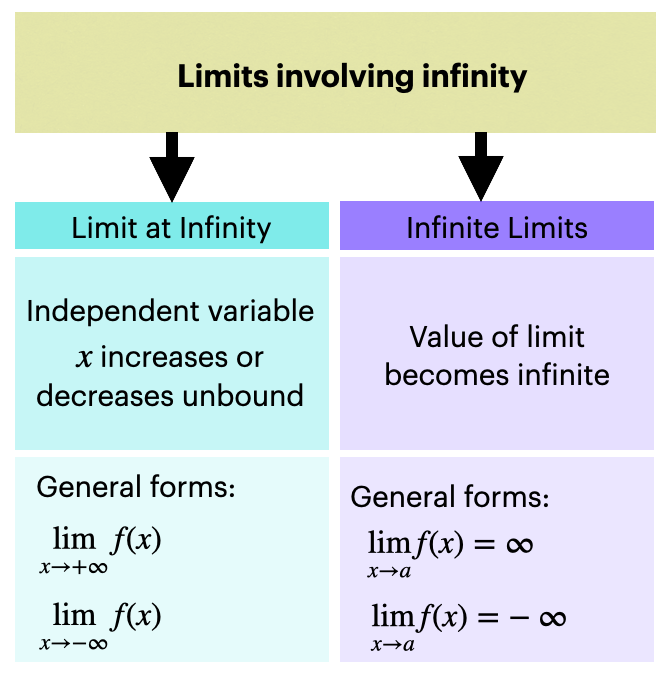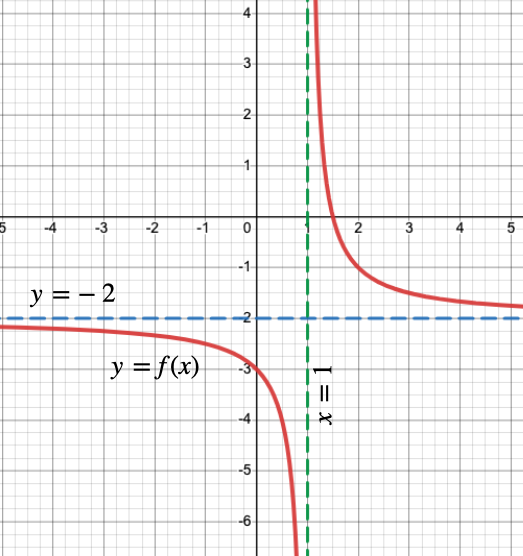407 493 6601

# Precalculus - Limits Involving Infinity

## Introduction

Sometimes while evaluating limits, we encounter the following two cases:

• Case $\left(i\right)$: The independent variable increases or decreases without bounds such as $\underset{x\to \infty }{\mathrm{lim}}f\left(x\right)$ and $\underset{x\to -\infty }{\mathrm{lim}}f\left(x\right)$. These limits are called limits at infinity.
• Case $\left(ii\right)$: The value of the limit of a function $f\left(x\right)$ becomes $\infty$ or $-\infty$ at any real value $a$.

These are collectively termed limits involving infinity. The figure shown summarizes the above discussion.## Horizontal and Vertical Asymptotes

Horizontal Asymptotes: Consider the two cases of limits mentioned below.

• $\underset{x\to \infty }{\mathrm{lim}}f\left(x\right)=L$: as $x$ "approaches" $\infty$$f\left(x\right)$ "approaches" a real value $L$.
• $\underset{x\to -\infty }{\mathrm{lim}}f\left(x\right)=M$: as $x$ "approaches" $-\infty$$f\left(x\right)$ "approaches" a real value $M$.

In all the above-mentioned cases, we can see that the independent variable reaches , the function reaches a real value , respectively. Hence, the horizontal line is called the horizontal asymptote of the function $f\left(x\right)$.

Vertical Asymptotes: Consider some of the cases of limits mentioned below.

• : as $x$ "approaches" $a$$f\left(x\right)$ "approaches" .
• : as $x$ "approaches" $a$ from the left, $f\left(x\right)$ "approaches" .
• : as $x$ "approaches" $a$ from the right, $f\left(x\right)$ "approaches" .

In all the above-mentioned cases, we can see that the function at $x=a$ either reaches . Hence, the vertical line $x=a$ is called the vertical asymptote of the function $f\left(x\right)$.In the graph shown above, $y=-2$ is the horizontal asymptote and $x=1$ is the vertical asymptote.

## Solved Examples

Example 1: Evaluate $\underset{x\to \infty }{\mathrm{lim}}\frac{3x-5}{2x+3}$.

Solution: $\underset{x\to \infty }{\mathrm{lim}}\frac{3x-5}{2x+3}$

$=\underset{x\to \infty }{\mathrm{lim}}\frac{x\left(3-\frac{5}{x}\right)}{x\left(2+\frac{3}{x}\right)}$

$=\underset{x\to \infty }{\mathrm{lim}}\frac{\left(3-\frac{5}{x}\right)}{\left(2+\frac{3}{x}\right)}$

$=\frac{\left(3-0\right)}{\left(2+0\right)}$

$=\frac{3}{2}$

Example 2: Evaluate $\underset{x\to -\infty }{\mathrm{lim}}\left(11-\frac{1}{x}\right)$.

Solution: $\underset{x\to -\infty }{\mathrm{lim}}\left(11-\frac{1}{x}\right)$

$=\underset{x\to -\infty }{\mathrm{lim}}\left(11\right)-\underset{x\to -\infty }{\mathrm{lim}}\left(\frac{1}{x}\right)$

$=11-0$

$=11$

## Cheat Sheet

• In a limit, if the independent variable approaches infinity or negative infinity, it is called a limit at infinity.
• Likewise, in a limit, if the function approaches infinity or negative infinity at some real value of the independent variable, it is termed an infinite limit.
• Collectively these two cases are called limits involving infinity.

## Blunder Areas

• The limit of a function at infinity can be a finite value as well as infinity.